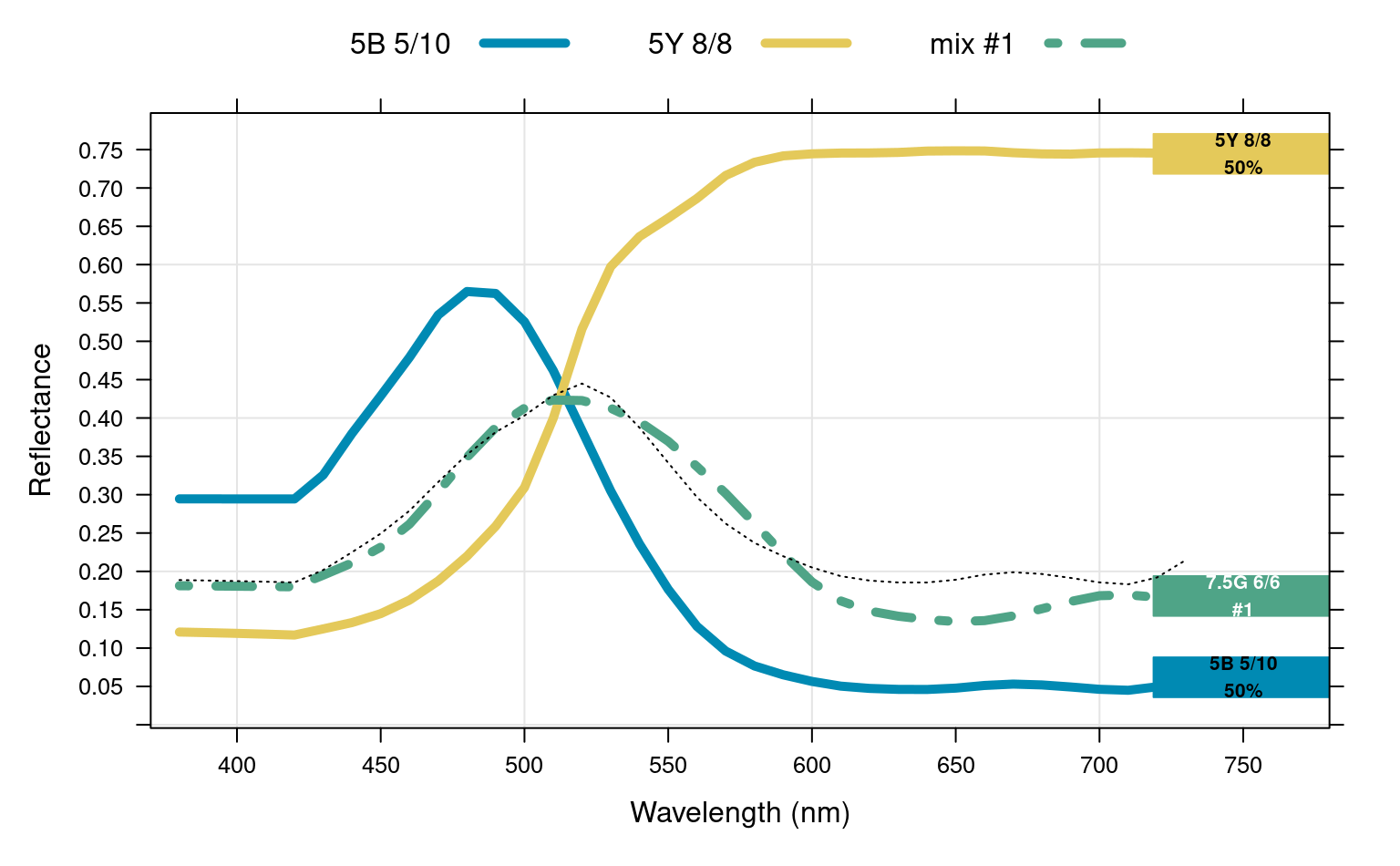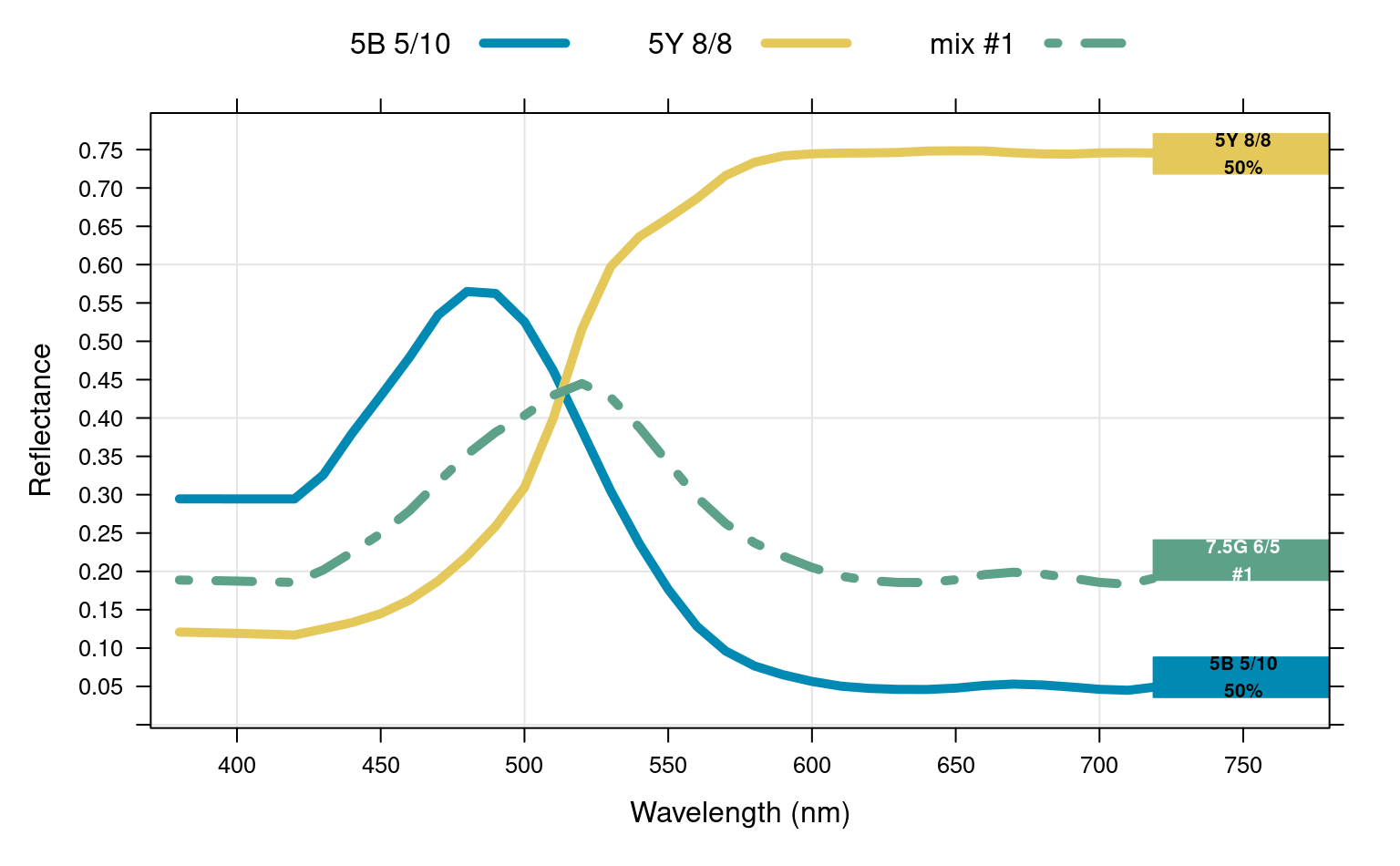Lattice visualization demonstrating subtractive mixtures of colors in Munsell notation and associated spectra.

plotColorMixture(
x,
w = rep(1, times = length(x))/length(x),
mixingMethod = c("reference", "exact"),
n = 1,
swatch.cex = 6,
label.cex = 0.85,
showMixedSpec = FALSE,
overlapFix = TRUE
)

## Arguments

x

vector of colors in Munsell notation, should not contain duplicates

w

vector of weights, can sum to any number

mixingMethod

approach used to simulate a mixture:

• reference : simulate a subtractive mixture of pigments, selecting n closest reference spectra

• exact: simulate a subtractive mixture of pigments, color conversion via CIE1931 color-matching functions (see mixMunsell)

n

number of closest mixture candidates when mixingMethod = 'reference' (see mixMunsell), results can be hard to interpret when n > 2

swatch.cex

scaling factor for color swatch

label.cex

scaling factor for swatch labels

showMixedSpec

show weighted geometric mean (mixed) spectra as dotted line (only when mixingMethod = 'reference')

overlapFix

attempt to "fix" overlapping chip labels via fixOverlap

## Value

a lattice graphics object

## Details

If present, names attribute of x is used for the figure legend.

D.E. Beaudette

## Examples


# color chips
chips <- c('5B 5/10', '5Y 8/8')
names(chips) <- chips

# weights
wt <- c(1, 1)

plotColorMixture(
x = chips,
w = wt,
swatch.cex = 4,
label.cex = 0.65,
showMixedSpec = TRUE,
mixingMethod = 'reference'
)plotColorMixture(
x = chips,
w = wt,
swatch.cex = 4,
label.cex = 0.65,
mixingMethod = 'exact'
)